#### Contrasts

For a testable hypothesis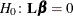, the Wald F statistic is computed as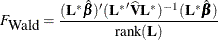whereis a contrast vector or matrix that you specify,is the vector of regression parameters,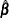is the estimated regression coefficients,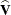is the estimated covariance matrix of, rank() is the rank of, and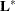is a matrix such that

•has the same number of columns as•has full row rank

• the rank ofequals the rank of thematrix

• all rows ofare estimable functions

• the Wald F statistic computed by using thematrix is equivalent to the Wald F statistic computed by using thematrix with any row deleted that is a linear combination of previous rows

Ifis a full-rank matrix and all rows ofare estimable functions, thenis the same as. It is possible thatmatrix cannot be constructed for a given set of linear contrasts, in which case the contrasts are not testable.

If the DF=NONE option in the MODEL statement is specified, then the procedure performs a chi-square significance test.